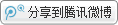用户名：
密码：###### 魏屹东 薛平：费泽尔认知规律及其哲学意蕴(一)心脑状态同一律

(z)(t)(B*zt= = ＞M*zt)

(二)心灵状态因果律(三)心灵认知刺激反应律

(a)：(z)(t)[Mzt= = ＞(Szt=u= ＞Rzt’)]
(b)：(z)(t)[Mzt= = ＞(Szt=p= ＞Rzt’)]

(四)心脑隐射同一律

(z)(t)(Bzt= = ＞Mzt)

(五)脑认知刺激反应律

(a)：(z)(t)[Bzt= = ＞(Szt=u= ＞Rzt’)]
(b)：(z)(t)[Bzt= = ＞(Szt=p= ＞Rzt’)]

(六)脑状态因果反应律

(a)：(z)(t)[B*zt= = ＞(EFzt=u= ＞SAzt’)]
(b)：(z)(t)[B*zt= = ＞(EFzt=p= ＞SAzt’)]

①詹姆士.费泽尔(James Herny Fetzer 1940－ )，美国哲学家，明尼苏达大学德鲁斯分校荣誉教授，在科学哲学、计算机科学、人工智能和认知科学方面颇有建树，发表了100多篇论文，出版了20多部相关领域的著作。

【参考文献】
James H. Fetzer. Philosophy and Cognitive Science [M]. New York: Paragon Issues in Philosophy, 1997.
斯马特：形而上学文集[C]//载尼克拉斯.布宁，余纪元．西方哲学英汉对照词典．北京：人民出版社，2001:469.
吉尔伯特.赖尔：心的概念[M].徐大建，译．北京：商务印书馆，1992:43.
彭孟尧．人心难测：心与认知的哲学问题[M].北京：生活.读书.新知三联书店，2006:48.
罗姆.哈瑞：认知科学导论[M].魏屹东，译．上海：上海科技教育出版社，2006:199－201.
（原载：《科学技术哲学研究》2012年3期。）ICP证号：浙ICP备16005704号-2